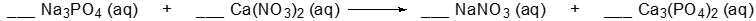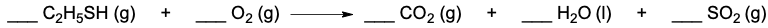Clutch Prep is now a part of Pearson
Ch.5 Classification & Balancing of Chemical ReactionsWorksheetSee all chapters

# Balancing Chemical Equations (Simplified)

See all sections
Sections
Chemical Reaction: Chemical Change
Law of Conservation of Mass
Balancing Chemical Equations (Simplified)
Solubility Rules
Molecular Equations
Types of Chemical Reactions
Complete Ionic Equations
Calculate Oxidation Numbers
Redox Reactions
Spontaneous Redox Reactions
Balancing Redox Reactions: Acidic Solutions
Balancing Redox Reactions: Basic Solutions
Balancing Redox Reactions (Simplified)
Galvanic Cell (Simplified)

Balancing Chemical Equations require the number of atoms to be the same on both sides of the arrow.

###### Balancing Chemical Equations

Concept #1: Balancing Chemical Equations

Example #1: Write the balanced equation for the following by inserting the correct coefficients in the blanks:

___ C4H10 (g) + ___ O2 (g) ___ H2O (l) + ___ CO2 (g)

Practice: Write the balanced equation for the following by inserting the correct coefficients in the blanks.Practice: Determine the total sum of the coefficients after balancing the following equation.